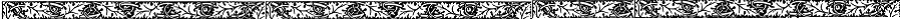# Famous Ancient Greek Mathematicians Facts

A discussion on famous ancient Greek people would be incomplete without information on the numerous famous ancient Greek mathematicians. In facts the word "mathematics" is derived from the ancient Greek word "mathema" which means "subject of instruction". Greek mathematics refers to mathematical theories developed in the Greek language from around 624 BC through the 4th century AD. Below is a list of many of these famous mathematicians along with facts about who they were and what their important contributions were.

## Interesting Facts about Ancient Greek Mathematicians

• Many historians believe that the early Greek mathematicians were influenced by and learned mathematical concepts from the ancient Egyptian civilization and Babylonian civilization.
• The classical period of Greek mathematics was followed by the Hellenistic period of mathematics. The Hellenistic period of ancient Greece began with the death of Alexander the Great in 323 BC. His conquest brought the Greek culture and language to the numerous regions he conquered. This period gave rise to Hellenistic mathematics where Greek mathematics merged with mathematics from other regions; mostly ancient Egypt and Babylon.

## Famous Ancient Greek Mathematicians List

### Thales (624 - 548 BC)

• He is one of the earliest Greek mathematicians and many historians regard him as the first true mathematician.
• Very little is known about Thales; including when he was born and when he died.
• He used geometry to solve problems including determining the height of ancient Egyptian pyramids based on the lengths of its shadows.
• The "Theorem of Thales" and the "Intercept theorem", an important theory in geometry, are attributed to him.

### Pythagoras (570 BC - 495 BC)

• Most of what we know about Pythagoras was recorded hundreds of years after his death and is therefore unreliable.
• He is best known for the theory that bears his name; the Pythagorean Theorem. This theory deals with the relationships between the three sides of a right triangle.
• There are no surviving texts written by this mathematician.

### Euclid (approximately 300 BC)

• Euclid (also called Euclid of Alexandria) is referred to as the father of geometry.
• Very little is known about his life; it is known he lived around 300 BC.
• He wrote a collection of books called "The Elements". These books were used up until the early 20th century as the main source for teaching mathematics; especially geometry.

### Archimedes (approximately 287 BC - 212 BC)

• Most historians rank Archimedes as one of the greatest mathematicians that ever lived.
• Many mathematicians believe Archimedes discovered calculus 1500 years before Isaac Newton and Gottfried Leibniz discovered it.
• Archimedes gave accurate approximation of pi which is the ratio of a circle's circumference to its diameter.

### Eratosthenes (276 BC - 195 BC)

• Not only was he a great mathematician but he is also famous for founding the discipline of geography. His other interest included poetry, music, and astronomy.
• This mathematician is perhaps most famous for calculating, with incredible accuracy, the circumference of the Earth.
• Eratosthenes accurately calculated both the tilt of the Earth's axis and the distance from the Earth to the Sun.
• He became the chief librarian at the famous Library of Alexandria.

### Hipparchus (190 BC – 120 BC)

• Hipparchus is considered the founder of trigonometry.
• He is perhaps best known for the discovery of precession of the equinoxes.